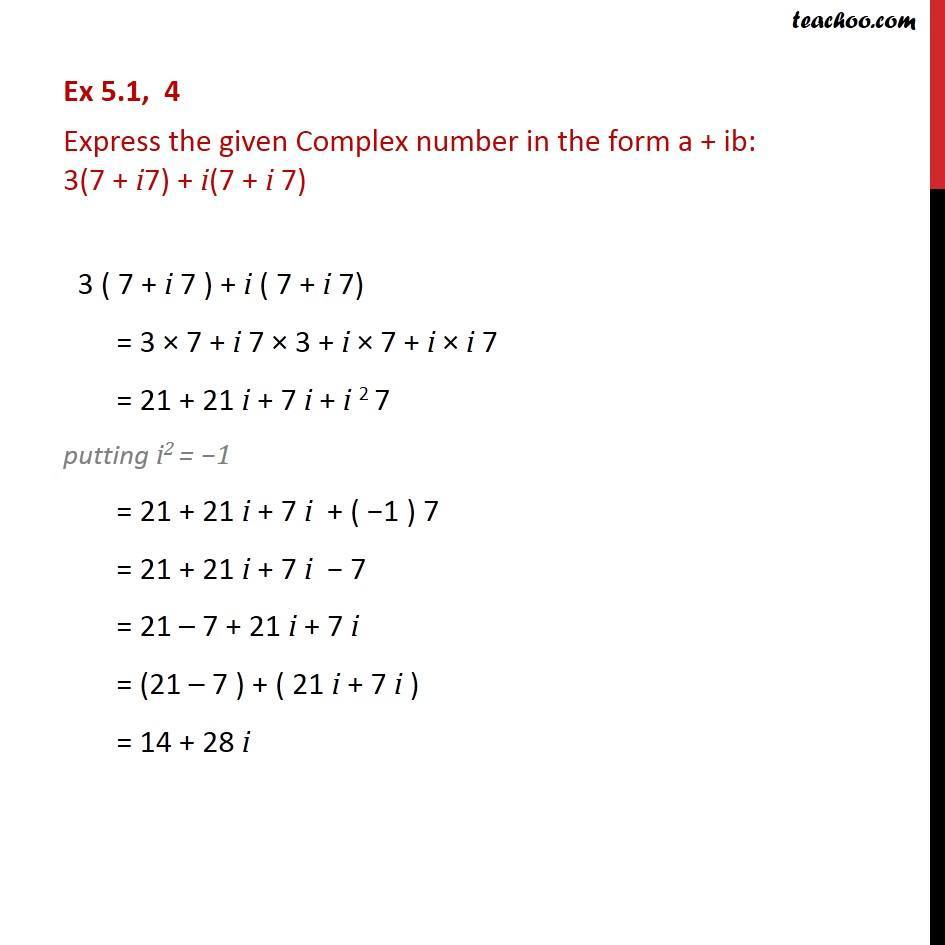Ex 5.1

Chapter 5 Class 11 Complex Numbers (Term 1)
Serial order wise### Transcript

Ex 5.1, 4 Express the given Complex number in the form a + ib: 3(7 + i7) + i(7 + i 7) 3 ( 7 + i 7 ) + i ( 7 + i 7) = 3 × 7 + i 7 × 3 + i × 7 + i × i 7 = 21 + 21 i + 7 i + i 2 7 putting i2 = −1 = 21 + 21 i + 7 i + ( −1 ) 7 = 21 + 21 i + 7 i − 7 = 21 – 7 + 21 i + 7 i = (21 – 7 ) + ( 21 i + 7 i ) = 14 + 28 i